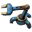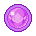## [posila] [0.17.59] Fog of war does not work

This subforum contains all the issues which we already resolved.
XaLpHa89
Fast InserterPosts: 119
Joined: Wed Jan 24, 2018 6:59 am
Contact:

### [posila] [0.17.59] Fog of war does not work

Only extracting raw materials from smelting furnaces of another force should be invisible.20190728195841_1.jpg (547.24 KiB) Viewed 1687 times
Last edited by XaLpHa89 on Tue Jul 30, 2019 10:38 pm, edited 1 time in total.

posila
Factorio StaffPosts: 5147
Joined: Thu Jun 11, 2015 1:35 pm
Contact:

### Re: [posila] [0.17.59] Fog of war does not work

Thanks for the report.

I think the inventory update notifications should not be visible to enemy forces at all, so I did that for 0.17.61

XaLpHa89
Fast InserterPosts: 119
Joined: Wed Jan 24, 2018 6:59 am
Contact:

### Re: [posila] [0.17.59] Fog of war does not work

I have the same problem with drawings. Or did I program it wrong?20190730234550_1.jpg (569.56 KiB) Viewed 1537 times

Code: Select all

``````    -- /silent-command circle_add( game.players[ 1 ] )

-- /silent-command circle_change( game.players[ 1 ] )

-- /silent-command circle_remove( game.players[ 1 ] )

function initialize_forces()

game.create_force( 'force_player_one' )

game.create_force( 'force_player_two' )

end

local table_of_colors = { squad = { r = 75, g = 155, b = 45 }, team = { r = 65, g = 120, b = 200 }, enemy = { r = 190, g = 55, b = 50 }, neutral = { r = 77, g = 77, b = 77 }, damage = { r = 255, g = 0, b = 255 }, white = { r = 255, g = 255, b = 255 } }

player.force = game.forces.force_player_one

if global.table_of_circles == nil then global.table_of_circles = {} end

if global.table_of_circles[ player.index ] == nil then

-- local one = rendering.draw_circle( { target = player.character, target_offset = { x = 0, y = - 2.5 }, force = game.forces.force_player_one, surface = player.surface, color = table_of_colors.team, radius = 0.2, filled = true, only_in_alt_mode = false } )

-- local two = rendering.draw_circle( { target = player.character, target_offset = { x = 1, y = - 2.5 }, force = game.forces.force_player_two, surface = player.surface, color = table_of_colors.enemy, radius = 0.2, filled = true, only_in_alt_mode = false } )

local one = rendering.draw_circle( { target = player.position, force = game.forces.force_player_one, surface = player.surface, color = table_of_colors.team, radius = 3, filled = true, only_in_alt_mode = false } )

local two = rendering.draw_circle( { target = { x = player.position.x + 80, y = player.position.y }, force = game.forces.force_player_two, surface = player.surface, color = table_of_colors.enemy, radius = 3, filled = true, only_in_alt_mode = false } )

global.table_of_circles[ player.index ] = { one = one, two = two }

end

end

function circle_change( player )

if global.table_of_circles[ player.index ] ~= nil then

local one = global.table_of_circles[ player.index ].one

local two = global.table_of_circles[ player.index ].two

end

end

function circle_remove( player )

if global.table_of_circles[ player.index ] ~= nil then

rendering.destroy( global.table_of_circles[ player.index ].one )

rendering.destroy( global.table_of_circles[ player.index ].two )

global.table_of_circles[ player.index ] = nil

end

end
``````

Rseding91
Factorio StaffPosts: 12224
Joined: Wed Jun 11, 2014 5:23 am
Contact:

### Re: [posila] [0.17.59] Fog of war does not work

The lua rendering API pays no attention to fogg of war. It just draws on the screen at all times.
If you want to get ahold of me I'm almost always on Discord.

XaLpHa89
Fast InserterPosts: 119
Joined: Wed Jan 24, 2018 6:59 am
Contact:

### Re: [posila] [0.17.59] Fog of war does not work

I want to portray friend and foe somehow. For example Symbolized with a colored circle. Does anyone have an idea how else to do it?